# RD Sharma Solutions Class 9 CooRDinate Geometry Exercise 11.1

## RD Sharma Solutions Class 9 Chapter 11 Ex 11.1

Q1) Plot the following points on the graph paper:

(i) (2,5)            (ii) (4,-3)          (iii) (-5,-7)        (iv) (7,-4)         (v) (-3,2)

(vi) (7,0)          (vii) (-4,0)        (viii) (0,7)        (ix) (0,-4)         (x) (0,0)

Solution:

The given points are,

A (2,5), B (4,-3), C (-5,-7), D (7,-4), E (-3,2),

F (7,0), G (-4,0), H (0,7), I (0,-4), J (0,0)

Let X ‘OX and Y ‘ OY be the coordinate axes.

(i) Here for the given point the abscissa is 2 units and ordinate is 5 units. The point is in the first quadrant. So it will look like as shown in the following figure.(ii) Here for the given point the abscissa is 4 units and ordinate is -3 units. The point is in the fourth quadrant. So it will look like as shown in the following figure.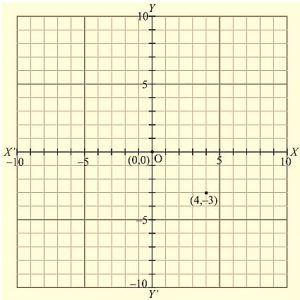(iii) Here for the given point the abscissa is -5 units and ordinate is -7 units. The point is in the third quadrant. So it will look like as shown in the following figure.(iv) Here for the given point the abscissa is 7 units and ordinate is -4 units. The point is in the fourth quadrant. So it will look like as shown in the following figure.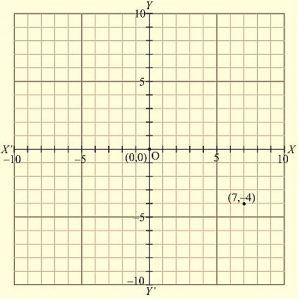(v) Here for the given point the abscissa is -3 units and ordinate is 2 units. The point is in the second quadrant. So it will look like as shown in the following figure.(vi) Here for the given point the abscissa is 7 units and ordinate is 0 units. The point is on the x-axis. So it will look like as shown in the following figure.(vii) Here for the given point the abscissa is -4 units and ordinate is 0 units. The point is on the x-axis. So it will look like as shown in the following figure.(viii) Here for the given point the abscissa is 0 units and ordinate is 7 units. The point is on the y-axis. So it will look like as shown in the following figure.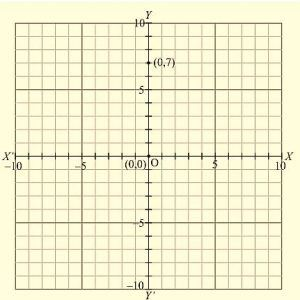(ix) Here for the given point the abscissa is 0 units and ordinate is -4 units. The point is on the y-axis. So it will look like as shown in the following figure.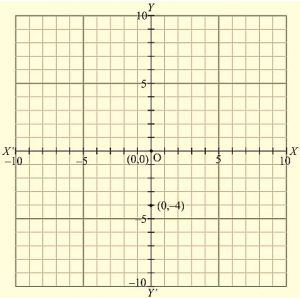(x) Here for the given point the abscissa is 0 units and ordinate is 0 units.

The point is basically intersection of the coordinate axis. So it will look like as shown in the following figure.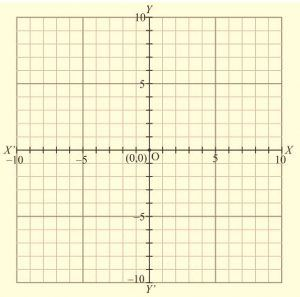Q2) Write the coordinates of each of the following points marked in the graph paper.Solution:

A (3,1), B (6,0), C (0,6), D (-3,0), E (-4,3), F (-2,-4), G (0,-5), H (3,-6), P (7,-3), Q (7,6)

1) The distance of point A from y-axis is 3 units and that of from x-axis is 1 units. Since A lies in the first quadrant, so its coordinates are (3,1).

2) The distance of point B from y-axis is 6 units and that of from x-axis is 0 units. Since B lies on x-axis, so its coordinates are (6,0).

3) The distance of point C from y-axis is 0 units and that of from x-axis is 6 units. Since C lies on y-axis, so its coordinates are (0,6).

4) The distance of point D from y-axis is -3 units and that of from x-axis is 0 units. Since D lies on x-axis, so its coordinates are (-3,0).

5) The distance of point E from y-axis is -4 units and that of from x-axis is 3 units. Since E lies in the second quadrant, so its coordinates are (-4,3).

6) The distance of point F from y-axis is -2 units and that of from x-axis is -4 units. Since F lies in the third quadrant, so its coordinates are (-2,-4).

7) The distance of point G from y-axis is 0 units and that of from x-axis is -5 units. Since G lies on y-axis, so its coordinates are (0,-5).

8) The distance of point H from y-axis is 3 units and that of from x-axis is -6 units. Since H lies in the fourth quadrant, so its coordinates are (3,-6).

9) The distance of point P from y-axis is 7 units and that of from x-axis is -3 units. Since P lies in the fourth quadrant, so its coordinates are (7,-3).

10) The distance of point Q from y-axis is 7 units and that of from x-axis is 6 units. Since Q lies in the first quadrant, so its coordinates are (7,6).